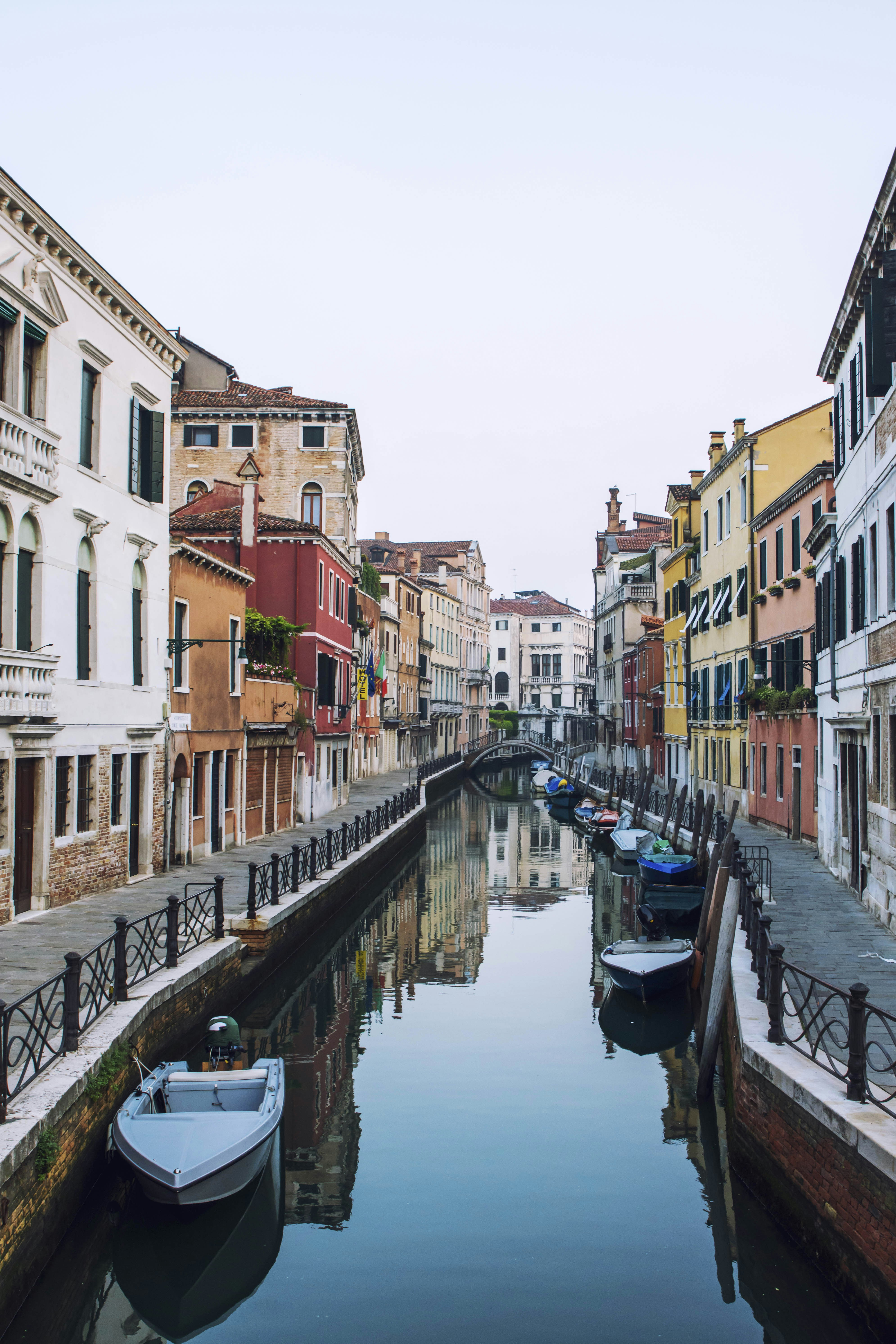HackerEarth - The colorful street Solution

# HackerEarth - The colorful street SolutionThere is a street by the name of a colorful street in Pretty Town. The residents of the house have decided that they will paint their houses in either Pink, Orange, or Yellow color and not other. They have also decided that no two adjacent houses will have the same color. For house i, i−1 and i+1 are the neighbors and note that the first and the last house are not neighbors.

The cost of painting a house in a particular color is different. The cost of painting the first house in color yellow may be different than what its for painting the second house in the same color.

You have to find the minimum cost of painting the houses which satisfy the given condition.

Input Constraints — Number of houses will contain between 1 and 20 elements, inclusive. — Each line of input for houses will have three values for the cost of coloring in each color. Each value will be between 1 and 1000.

Input Format — The first line will contain the number of test cases — T. — The second line will contain an integer N that will specify how many houses are there. — Each of the following N lines will contain 3 numbers separated by space that represents the cost of painting that house in either pink, orange, or yellow color.

Output Format Print T lines showing the cost of painting the houses for each test case.

SAMPLE INPUT

1
2
11 12 13
14 15 16

SAMPLE OUTPUT

26

Explanation

The first house should be painted in pink (11), the second should be painted in orange (15). So the total cost becomes 11+15=26

### Solution in Python

def minCost(costs):
for i in range(1,len(costs)):
x,y = costs[i],costs[i-1]
x += min(y, y)
x += min(y, y)
x += min(y, y)
return min(costs[-1])
t = int(input())
for _ in range(t):
n = int(input())
costs = [list(map(int,input().strip().split())) for i in range(n)]
print(minCost(costs))